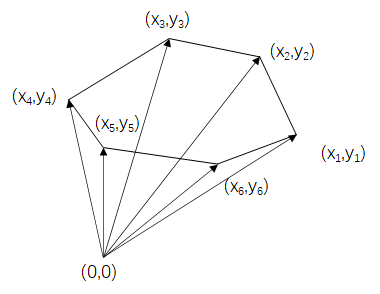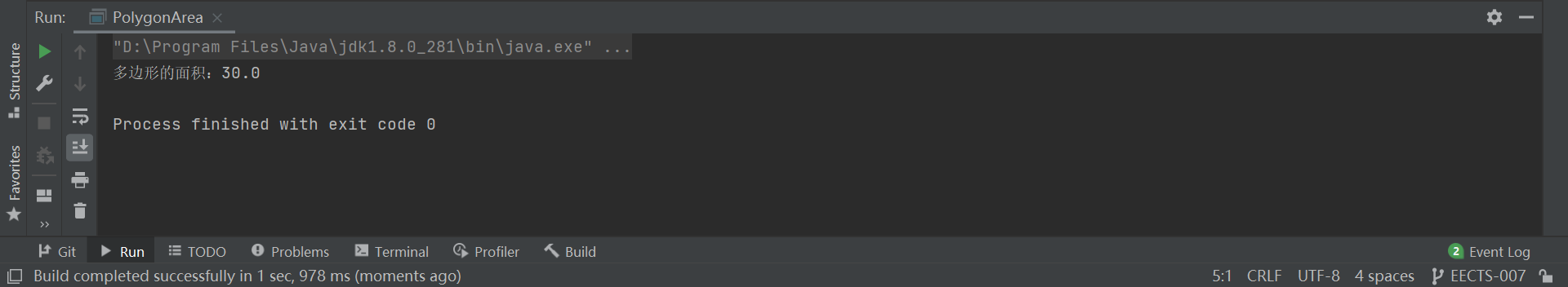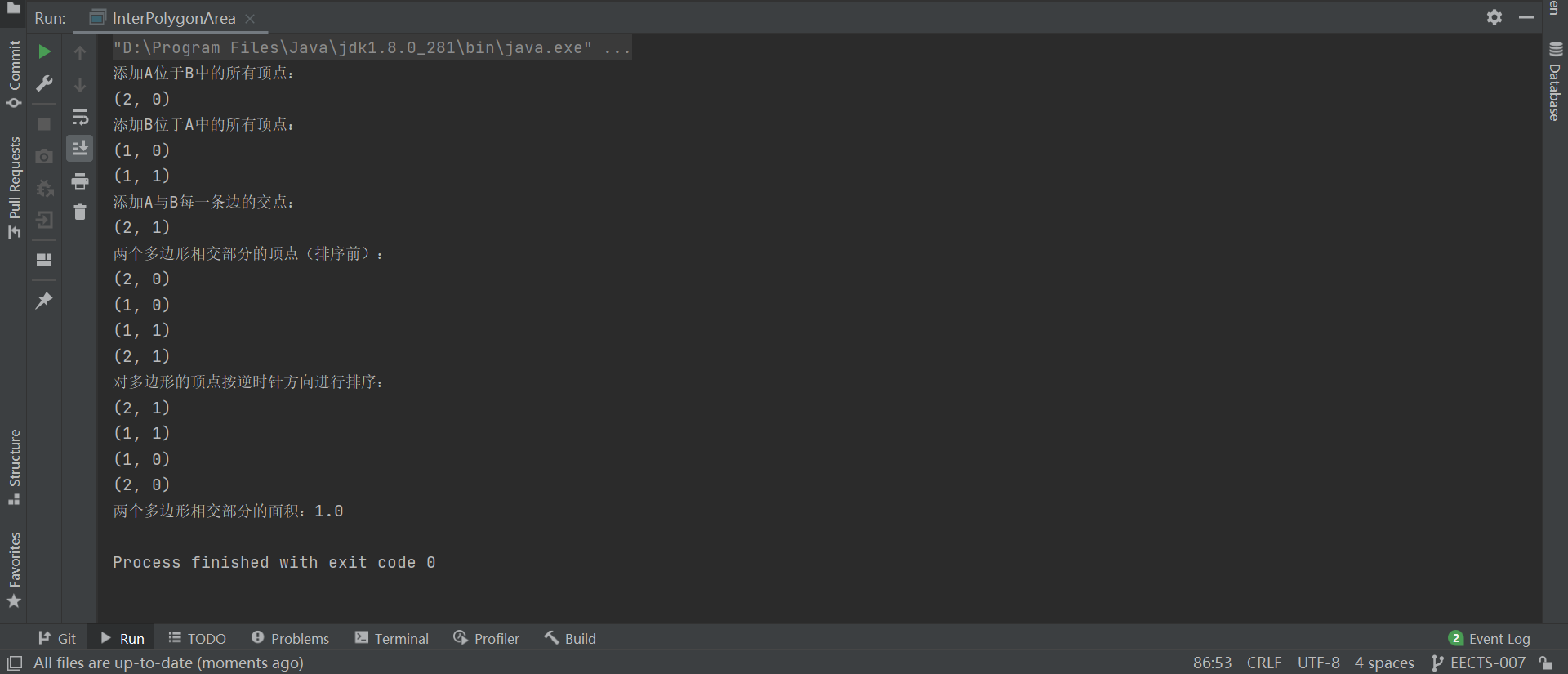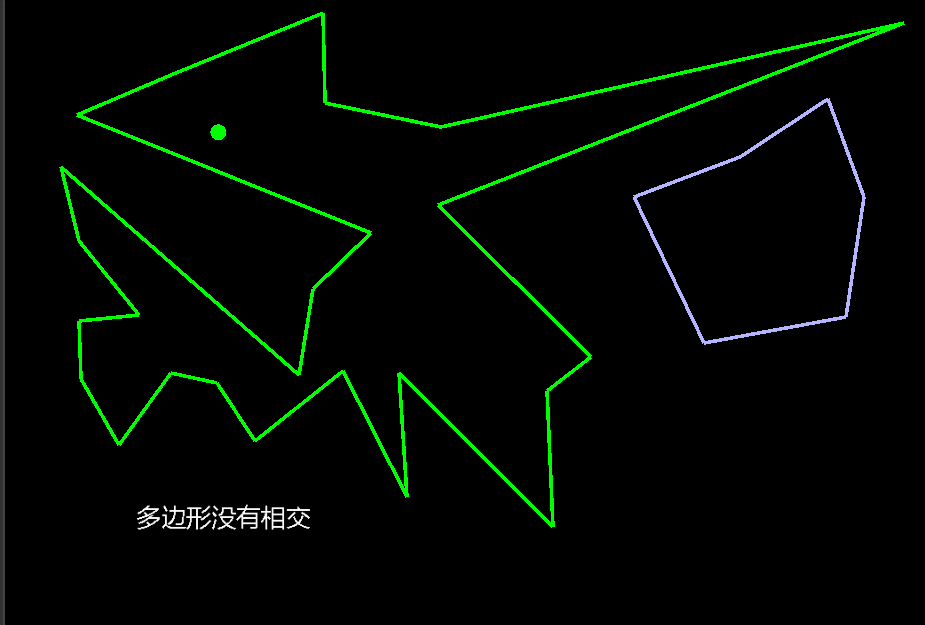• 2019-01-08 14:40:45

## 前言

继续总结关于图形学的问题，这次总结下多边行的相交问题，同样可以自行阅读参考文献了解，以下是本人的自行总结内容。

参考文献:《计算机图形学——用OpenGL实现(第2版)》 清华大学出版社

## 平面直线与多边形相交

首先要说明一个定义——外法向量，指的是多边形的边指向多边形外部的法向量。要想确立多边形每条边的外法向量，简单的方法就是顺时针记录多边形的顶点集合 P i P_i ，对应边的集合 E i = P i + 1 − P i E_i = P_{i+1} - P_i ，边的外法向量集合 E i ⊥ E^\perp_i 便是 E i E_i 的逆时针法向量
具体步骤便是，依次对多边形的每一条边做直线与线段的相交检测（具体请参考前面的章节平面图形原理总结（1）：直线相交

## 平面线段与多边形相交

步骤大致与直线与多边形相交的方法相似，可用两直线相交的方法，但是要记录下所有线段所在直线与多边形边所在直线的相交点的t值，同时要区分入点与出点。出入点区分方法是用线段的向量 n ⃗ \vec n 与多边形边的外法向量 E i ⊥ E^\perp_i 做点乘：
n ⃗ ⋅ E i ⊥ &lt; 0 \vec n \cdot E^\perp_i &lt; 0 ，则为入点；
n ⃗ ⋅ E i ⊥ &gt; 0 \vec n \cdot E^\perp_i &gt; 0 ，则为出点；
n ⃗ ⋅ E i ⊥ = 0 \vec n \cdot E^\perp_i = 0 ，则线段与多边行的边平行（考虑重合问题）；
取入点所有记录t与0的最大值，出点所有记录t与1的最小值；
若存在 0 ≤ t ≤ 1 0 \le t \le 1 ，则线段与多边形相交。

## 平面多边形与多边形相交

以上述线段与多边形相交为单元步骤，对多边形的每一条边做与另一多边形的相交判断，即可得到多边形与多边形的相交检测。

## 平面圆与多边形相交

具体步骤便是，依次对多边形的每一条边做圆与线段的相交检测（具体请参考前面的章节平面图形原理总结（2）：点与直线

## 补充：凸多边形的投影法相交检测

具体步骤为：检测图形对多边形每条边的法向量形成的坐标系做投影，若其中一边的检测中，存在图形的投影与多边形自身的投影范围没有重合部分，则图形与多边形不相交；反之，则相交。
注意：投影法只适用于凸多边形，凹多边形不适用。
【投影法没有参考书籍中记载，具体描述，可自行上网查找】

更多相关内容
• 简单多边形的相交、合并算法。...这个demo程序只是介绍了多边形相交、合并的算法，针对简单凹凸多边形可以正常处理。算法仅供参考！ 如需要支持内部有环的复杂多边形相交合并，请使用boost::polygon。
• 本资源对应的博文地址：https://blog.csdn.net/StevenKyleLee/article/details/88075814
• 原文地址 http://www.cnblogs.com/topcss/p/3575248.html ，基于该作者的代码基础上进行简单修改，使其在openlayers3上可用，有兴趣可以去看一下
• 保护私有信息的计算几何是一类特殊的安全多方计算问题，在军事、商业等领域具有重要的应用前景。...基于该协议，提出保护私有信息的两多边形相交面积计算协议；分析和证明上述协议的正确性、安全性和复杂性。
• 计算多边形的面积 设多边形各顶点的坐标为：(x1,y1),(x2,y2),(x3,y3)……(xn,yn)(x_1,y_1),(x_2,y_2),(x_3,y_3)……(x_n,y_n)(x1​,y1​),(x2​,y2​),(x3​,y3​)……(xn​,yn​) 则该多边形的面积为： S=12∑n=1∞...

### 计算多边形的面积

设多边形各顶点的坐标为：(x1, y1), (x2, y2), (x3, y3)……(xn, yn)，则该多边形的面积为：
S = 1 2 ∑ n = 1 ∞ ( x n y n + 1 − x n + 1 y n ) S = 1 2 [ ( x 1 y 2 − x 2 y 1 ) + ( x 2 y 3 − x 3 y 2 ) + … … + ( x n y 1 − x 1 y n ) ] S=\frac 12 \sum_{n=1}^{\infty} {(x_{n}y_{n+1}-x_{n+1}y_{n})} \\ S=\frac 12 [(x_1y_2-x_2y_1)+(x_2y_3-x_3y_2)+……+(x_ny_1-x_1y_n)]多边形各顶点与原点相连，构成多个三角形，将所有三角形的面积相加或相减，得到的结果就是多边形的面积。

• 三角形的面积由两个向量的向量积的模计算得到： 1 2 ∣ ( x n y n + 1 − x n + 1 y n ) ∣ \frac 12 |(x_{n}y_{n+1}-x_{n+1}y_{n})|
• 面积相加或相减由向量积的方向确定： 1 2 ( x n y n + 1 − x n + 1 y n ) \frac 12 (x_{n}y_{n+1}-x_{n+1}y_{n})
package Polygon;

import java.awt.*;

/**
* 计算多边形的面积
*/
public class PolygonArea {

public static void main(String[] args) {

Polygon polygon = new Polygon(new int[]{3,5,12,9,5}, new int[]{4,11,8,5,6}, 5);
// 计算多边形的面积
PolygonArea polygonArea = new PolygonArea();
System.out.println("多边形的面积：" + polygonArea.getArea(polygon));
}

/**
* 计算多边形的面积
*/
public double getArea(Polygon polygon) {
double Area = 0;  // 多边形的面积
Integer Num = polygon.npoints;  // 顶点个数
// 添加第一个顶点到末尾
// 计算多边形的面积
for (int i = 0; i < Num; i++) {
Area += 0.5 * ((polygon.xpoints[i] * polygon.ypoints[i+1]) - (polygon.ypoints[i] * polygon.xpoints[i+1]));
}
return Math.abs(Area);
}
}### 计算两个多边形相交部分的面积

给出多边形PolygonA和多边形PolygonB，求两个多边形相交部分的面积。具体步骤如下：

• 添加A位于B中的所有顶点：循环A的所有顶点，判断是否位于B中。
• 添加B位于A中的所有顶点：循环B的所有顶点，判断是否位于A中。
• 添加A与B每一条边的交点：判断边与边是否相交，相交则求交点。
• 对多边形的顶点按逆时针方向进行排序。
• 计算多边形InterPolygon的面积。
package Polygon;

import java.awt.*;
import java.awt.geom.Line2D;

import static java.lang.Math.max;
import static java.lang.Math.min;

/**
* 计算两个多边形相交部分的面积
*/
public class InterPolygonArea {

public static void main(String[] args) {

Polygon polygonA = new Polygon(new int[]{0,2,2,0}, new int[]{0,0,2,2}, 4);
Polygon polygonB = new Polygon(new int[]{1,3,3,1}, new int[]{0,0,1,1}, 4);
// 计算两个多边形相交部分的面积
InterPolygonArea interPolygonArea = new InterPolygonArea();
System.out.println("两个多边形相交部分的面积：" + interPolygonArea.getInterArea(polygonA, polygonB));
}

/**
* 计算两个多边形相交部分的面积
*/
public double getInterArea(Polygon polygon1, Polygon polygon2) {
Polygon InterPolygon = new Polygon();  // 两个多边形相交部分
Integer Num1 = polygon1.npoints;  // 多边形A的顶点个数
Integer Num2 = polygon2.npoints;  // 多边形B的顶点个数

// 添加A位于B中的所有顶点：循环A的所有顶点，判断是否位于B中
System.out.println("添加A位于B中的所有顶点：");
for (int i = 0; i < Num1; i++) {
if(polygon2.contains(polygon1.xpoints[i], polygon1.ypoints[i])) {
System.out.println("(" + polygon1.xpoints[i] + ", " + polygon1.ypoints[i] + ")");
}
}

// 添加B位于A中的所有顶点：循环B的所有顶点，判断是否位于A中
System.out.println("添加B位于A中的所有顶点：");
for (int i = 0; i < Num2; i++) {
if(polygon1.contains(polygon2.xpoints[i], polygon2.ypoints[i])) {
System.out.println("(" + polygon2.xpoints[i] + ", " + polygon2.ypoints[i] + ")");
}
}

// 添加第一个顶点到末尾
// 添加A与B每一条边的交点：判断边与边是否相交，相交则求交点
System.out.println("添加A与B每一条边的交点：");
boolean flag = false;  // false为不相交，true为相交
for (int i = 0; i < Num1; i++) {
for (int j = 0; j < Num2; j++) {
// 判断多边形A的边(x1,y1)→(x2,y2)和多边形B的边(x3,y3)→(x4,y4)是否相交
double x1 = polygon1.xpoints[i], y1 = polygon1.ypoints[i];
double x2 = polygon1.xpoints[i+1], y2 = polygon1.ypoints[i+1];
double x3 = polygon2.xpoints[j], y3 = polygon2.ypoints[j];
double x4 = polygon2.xpoints[j+1], y4 = polygon2.ypoints[j+1];
// flag = Line2D.linesIntersect(x1, y1, x2, y2, x3, y3, x4, y4);
flag = isInterLines(x1, y1, x2, y2, x3, y3, x4, y4);
// 相交则求交点，并添加
if (flag) {
Point interSection = getInterSection(x1, y1, x2, y2, x3, y3, x4, y4);
System.out.println("(" + interSection.x + ", " + interSection.y + ")");
}
}
}

System.out.println("两个多边形相交部分的顶点（排序前）：");
for (int i = 0; i < InterPolygon.npoints; i++) {
System.out.println("(" + InterPolygon.xpoints[i] + ", " + InterPolygon.ypoints[i] + ")");
}

// 对多边形的顶点按逆时针方向进行排序
PolygonSort polygonSort = new PolygonSort();
Polygon interPolygon = polygonSort.PolygonClockwiseSort(InterPolygon);
System.out.println("对多边形的顶点按逆时针方向进行排序：");
for (int i = 0; i < interPolygon.npoints; i++) {
System.out.println("(" + interPolygon.xpoints[i] + ", " + interPolygon.ypoints[i] + ")");
}

// 计算两个多边形相交部分的面积
PolygonArea polygonArea = new PolygonArea();
return polygonArea.getArea(interPolygon);
}

/**
* 判断两个线段是否相交
*/
public boolean isInterLines(double x1, double y1, double x2, double y2, double x3, double y3, double x4, double y4) {
// 快速排斥实验：判断两条线段在x以及y坐标的投影是否有重合
if (max(x3, x4) < min(x1, x2) || max(x1, x2) < min(x3, x4)
|| max(y3, y4) < min(y1, y2) || max(y1, y2) < min(y3, y4)){
return false;
}
else {
// 跨立实验（不考虑线段端点作为交点）：点A和点B分别在线段CD两侧，点C和点D分别在线段AB两侧
// 满足条件：(A-B)×(B−D)∗(A−B)×(C−B)<0 && (A−D)×(C−D)∗(B−D)×(C−D)<0
if (((x2-x1)*(y4-y2) - (x4-x2)*(y2-y1)) * ((x2-x1)*(y3-y2) - (x3-x2)*(y2-y1)) < 0
&& ((x4-x1)*(y4-y3) - (x4-x3)*(y4-y1)) * ((x4-x2)*(y4-y3) - (x4-x3)*(y4-y2)) < 0) {
return true;
}
return false;
}
}

/**
* 计算两个相交线段的交点
*/
public Point getInterSection(double x1, double y1, double x2, double y2, double x3, double y3, double x4, double y4) {
double b1 = (y2 - y1) * x1 + (x1 - x2) * y1;
double b2 = (y4 - y3) * x3 + (x3 - x4) * y3;
double D = (x2 - x1) * (y4 - y3) - (x4 - x3) * (y2 - y1);
double D1 = b2 * (x2 - x1) - b1 * (x4 - x3);
double D2 = b2 * (y2 - y1) - b1 * (y4 - y3);
Point point = new Point();
point.setLocation(D1 / D, D2 / D);
return point;
}
}参考：

• https://blog.csdn.net/sfw673306004/article/details/103801376
展开全文• 暴力： 貌似只适用于求两个多边形相交面积，但是可以求任意多边形（凹凸）。 1.设两个多边形点集为P1，P2，相交区域点集为P。 2.遍历P1的点是否在P2内，若在内部，则加入点集P；对P2也做相同操作。 3.遍历P1的边和...

暴力： 貌似只适用于求两个多边形的相交面积，但是可以求任意多边形（凹凸）。
1.设两个多边形点集为P1P2，相交区域点集为P
2.遍历P1的点是否在P2内，若在内部，则加入点集P；对P2也做相同操作。
3.遍历P1的边和P2的边，求出两个多边形的交点，将交点加入P。
4.求点集P的面积。

半平面交： 这个只适用凸包，但是可以求任意个凸包最终相交的面积。
1.把每个凸包的点按照逆时针排序。（当然也看自己的算法是要什么顺序，大多数需要逆时针）
2.将每个凸包的边放入边集L。
3.求边集L的半平面交，再求半平面交的面积。
求一次半平面交：O(nlogn)，n为边数

题目：ECNU 1624

暴力就不写了，写个半平面交的：

#include<bits/stdc++.h>
#define ll long long
#define lf double
#define IOS std::ios::sync_with_stdio(false);cin.tie(0);cout.tie(0);
#define Rep(i,l,r) for(int i=(l);i<=(r);i++)
using namespace std;

struct Point {double x, y;};
typedef Point vector_t;
typedef Point point_t;
struct Line {Point x;vector_t v;};

double Cross(const vector_t& x, const vector_t& y) {return x.x * y.y - x.y * y.x;}  //叉积
vector_t operator*(const vector_t& v, double t) {return (vector_t){v.x * t, v.y * t};}
vector_t operator+(const vector_t& a, const vector_t& b) {return (vector_t){a.x + b.x, a.y + b.y};}
Point operator-(const Point& a, const Point& b) {return (Point){a.x - b.x, a.y - b.y};}

point_t GetLineIntersection(Line a,Line b){//求两直线交点
Point P=a.x;vector_t v=a.v;
Point Q=b.x;vector_t w=b.v;
vector_t u = P-Q;
double t = Cross(w, u)/Cross(v, w);
return P+v*t;//由直线的点向式而来
}

double eps=1e-6;
Point tmpP;
Line tmpL;
bool cmp1(const Line& a,const Line& b) { return atan2(a.v.y,a.v.x)<atan2(b.v.y,b.v.x); }//极角排序
int dcmp(double x){ if(fabs(x)<eps)return 0; if(x>0)return 1; return -1;}
bool Onleft(Line l,Point p) { return dcmp(Cross(l.v,(p-l.x))) > 0; }//ToLeftTest
int HalfPol(Line *l,int n,vector<Point> &p)
{
sort(l,l+n,cmp1);//把边按极角排序
int hd = 0,tl = 0;
tmpL = l;
for(int i=0;i<n;i++)
{
while(hd<tl&&!Onleft(l[i],tmpP[tl-1]))tl--;
while(hd<tl&&!Onleft(l[i],tmpP[hd]))hd++;
tmpL[++tl] = l[i];
if(!dcmp(Cross(tmpL[tl].v,tmpL[tl-1].v)))
{
tl--;
if(Onleft(tmpL[tl],l[i].x))tmpL[tl]=l[i];
}
if(hd<tl)tmpP[tl-1] = GetLineIntersection(tmpL[tl-1],tmpL[tl]);//求两直线交点
}
while(hd<tl&&!Onleft(tmpL[hd],tmpP[tl-1]))tl--;
if(tl-hd<=1)return 0;
tmpP[tl] = GetLineIntersection(tmpL[hd],tmpL[tl]);
for(int i=hd;i<=tl;i++)p.push_back(tmpP[i]);
return tl-hd+1;
}
double PolygonArea(point_t* p, int n){//p为端点集合，n为端点个数
double s = 0;
for(int i = 1; i < n-1; i++)
s += Cross(p[i]-p, p[i+1]-p);
return s/2;
}

int n,m;
Point P;
Line L;
vector<Point>s;
Point ss;

int main()
{
cin>>n;
int cntl=0;
Rep(i,0,n-1){
double x,y;cin>>x>>y;
P[i].x=x;P[i].y=y;
}
for(int i=0;i<n;i++){
L[cntl++].v=P[i]-P[(i+1)%n];
L[cntl].x=P[(i+1)%n];
}
cin>>m;
Rep(i,0,m-1){
double x,y;cin>>x>>y;
P[i].x=x;P[i].y=y;
}
for(int i=0;i<m;i++){//题目按顺时针给的,就反着来
L[cntl].v=P[i]-P[(i+1)%m];
L[cntl].x=P[(i+1)%m];
cntl++;
}
int sn=HalfPol(L,cntl,s);
std::copy(s.begin(),s.end(),ss);//把s的数据copy到ss
double S=PolygonArea(ss,sn);
printf("%.2lf\n",S);
return 0;
}


参考：ECNU 1624 求交集多边形面积
ECNU 1624 求交集多边形面积

展开全文算法
• ## 判断2个多边形相交

千次阅读 2020-03-06 15:21:32
2个多边形的边是否相交。 点在内部。2个多边形的顶点是否在另一个多边形的内部。 关于这2个条件的判断： 《碰撞检测：判断点是否在多边形内部》 https://blog.csdn.net/StevenKyleLee/article/details/88044589 ...需要判断2个条件

边相交。2个多边形的边是否相交。
点在内部。2个多边形的顶点是否在另一个多边形的内部。
关于这2个条件的判断：
《碰撞检测：判断点是否在多边形内部》
https://blog.csdn.net/StevenKyleLee/article/details/88044589
《碰撞检测：判断线段相交》
https://blog.csdn.net/StevenKyleLee/article/details/87934320

源码工程下载：

展开全文• 那么，判断f1和f2是否相交，可以将每条边用解析式表示，将两个多边形的边的解析式联立算交点，并将交点与解析式的取值范围相匹配，即可解决此问题。 解析式为y=kx+b，两点为(x1,y1)，(x2,y2)，那么 k=y1−y2x1−x2k...
• 可移动多边形，可改变多边形形状、顶点数量，最主要的是检测相交情况。
• 测绘地理信息技术专业教学资源库 PAGE - 4 - 多边形的相交 1实验目的 熟练掌握ArcGIS10多边形相交操作 掌握ArcGIS10中矢量数据相交的基本方法 2实验背景 相交被定义为进行多边形叠合输出层为保留原来两个输入图层的...
• 最近开发过程中遇到了这个问题，费了好大的劲才搞定，贴出来跟大家分享 假设lpVertex1[]里存放着多边形1的顶点，nVertexCount1为多边形1的顶点数 lpVertex2[]里存放着多边形2的顶点，nVertexCount2为多边形2的顶点数...
• 任意两个凸多边形A,B的相交区域面积计算，这里采用如下两步进行计算： 一、 计算相交区域对应的多边形，记为intersect 大体分为下面几个步骤： 1.记录A位于B中的所有顶点 2.记录B位于A中的所有顶点 3.记录A与B每一条...
• 用vc + mapobjects开发的源代码，用鼠标对多边形进行操作，实现了多边形相交计算功能。
• ypoints - an array of Y coordinates 所有点的y坐标 npoints - the total number of points in the Polygon 总共的点数 scene: 把商家通过地图拖拽的多边形各点传入进来，实例化Polygon对象 public boolean ...
• 本文记录一些常见的相交判断方法，属于游戏开发中比较常见的几何应用。 目录 一、线段之间的相交判断： 二、顶点走向判断 三、路径导航 四、顶点与凸包相交计算 五、线段与凸包的相交计算 六、凸包与凸包的...
• 这个开源库基于Unity开发，主要实现了凹多边形之间的相交判断，当然也可以应用于顶点与凹凸包、线段与凹凸包、凸包与凸包之间的相交判断，也就是说，这个方案基本可以解决前面两则文章中的所有多边形判断，效率也...
• 两个多边形相交区域面积求解的算法网上找到的有些层次不齐，但算法都大致相同，就是计算多边形线段相交求取交点，然后找到交叠区域。在查找算法和代码中发现一些好的程序，一并整理在此。 1、...
• 最近在做项目时遇到需要对二维的多边形进行相交及合并的操作，自己也写了很多方法，但是效果都不是很理想，最终决定使用GPC库，此库中有很多复杂的多边形裁剪方法，现对此库的使用方法做简单描述，源码在文末贴出。...c#
• 演示demo： 需要判断2个条件 边相交。2个多边形的边是否相交。 点在内部。2个多边形的顶点是否在另一个多边形的内部。 关于这2个条件的判断： 《碰撞检测：判断点是否在多边形内部》 ...
• 我通过将地图兼容的多边形转换为Polygon2d(http://geom-java.sourceforge.net/api/math/geom2d/polygon/package-summary.html)并使用相交方法来找到相交多边形的顶点列表来实现这一点。问题:除了两个相同的多边形...
• 之前基本上遇到的多边形切割问题都是凸多边形问题，而针对凹多边形的切割问题却很少。偶然发现一个做得特别棒的滑动切割的游戏，游戏中主要是使用多边形切割以及多边形碰撞算法。针对多边形切割的实现做了一下研究，...
• fabsf(d) 表示edge与要测试的这条线平行， n 表示edge在diff的右边，则表示没机会相交了， 如果>=0 也就是有可能与多边形相交，continue t = n / d; d说明dir在edge的右边 也就是说明dir是向着多边形里面来的 总之...
• 包含（相交的一种，交集为面积较小多边形） 相等（相交的一种，交集为 2 多边形本身） 相离（无交集） 先向大家阐述我的应用场景：需求方欲通过在地图上绘制蜂窝以分配员工所负责区域。纯手工绘制易将道路、...
• 这是用JS编写的多边形相交算法的演示。 基于提供的模板的项目的可视部分。 可以通过GitHub Pages获得演示站点，网址为：[vrd.github.io/js-intersect]（ ）。 项目包含以下文件： index.html-演示页面 test.html-...JavaScript
• 自己用VC++作的多边形程序，包括求面积、凹凸性、凸包、两多边形相交等。
• // check if a intersects b // a中相邻2个点组成的线段和b多边形相交 for ( i = 0, l = a.length; i ; ++i ) { var a1 = a[i]; var a2 = a[(i+1)%l]; if ( cc.Intersection.linePolygon( a1, a2, b ) ) return ...cocos
• 基于GPU的任意多边形相交面积计算方法高艺1,2,罗健欣1,裘杭萍1,唐斌1,吴波1【摘要】一直以来，任意多边形相交面积的高效计算都是地理信息系统中空间分析算法研究的重点。文中提出了一种基于GPU的栅格化多边形相交......